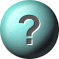Moles and Calculations in Chemistry Quiz - gcsescience.com.1. A mole is defined asa) An abbreviation for molecule b) The amount of nitrogen in one litre of air c) The amount of energy needed to          heat 1g of platinum through 1°C d) The number of atoms in 12g of carbon twelve2. What is the mass of one mole of oxygen molecules?        Oxygen is O2.  RAM of oxygen = 16.a) 8g b) 16g c) 32g d) 64g3. What is the RFM of CaCO3? Ca = 40. C = 12. O = 16.a) 68 b) 100 c) 124 d) 2044. What is the mass of one mole of CaCO3?        Ca = 40. C = 12. O = 16.a) 68g b) 100g c) 124g d) 132g5. What is the percentage of nitrogen in NH4NO3?        N = 14. H = 1. O = 16.a) 17.5% b) 29% c) 35% d) 58%6. 33.6g of iron reacts with 14.4g of oxygen. What is the      empirical formula of iron oxide?a) FeO b) Fe2O c) FeO2 d) Fe2O37. A current of 10 Amps is passed for 5 hours through molten       aluminium oxide. What mass of aluminium is deposited?a) 0.622g b) 1.865g c) 16.79g d) 27.0g

This is more feedback!
This is the feedback!
GCSE Chemistry         GCSE Physics         All Chemistry Quizzes
Science Practice Worksheets & Activities  |  Online Self-Paced Science Lessons  |  Free Test Maker